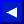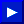EXAMPLES of FN Use:
```   40 PRINT FN A(9)
50 R=FN AA(9)
60 G=G+FN A9(10)
```

In line 50 above, the number 9 inside the parentheses does not affect the outcome of the function, because the function definition in line 20 doesn't use the variable in the parentheses. The result is Y times Z, regardless of the value of X. In the other two functions, the value in parentheses does affect the result.

### DIM

TYPE: Statement
FORMAT: DIM <variable> ( <subscripts> )[, <variable> ( <subscripts> )...]

Action: This statement defines an array or matrix of variables. This allows you to use the variable name with a subscript. The subscript points to the element being used. The lowest element number in an array is zero, and the highest is the number given in the DIM statement, which has a maximum of 32767.

The DIM statement must be executed once and only once for each array. A REDIM'D ARRAY error occurs if this line is re-executed. Therefore, most programs perform all DIM operations at the very beginning.

There may be any number of dimensions and 255 subscripts in an array, limited only by the amount of RAM memory which is available to hold the variables. The array may be mode up of normal numeric variables, as shown above, or of strings or integer numbers. If the variables are other than normal numeric, use the \$ or % signs after the variable name to indicate string or integer variables,This page has been created by Sami Rautiainen. Read the small print. Last updated November 14, 1998.